Analytical Mechanics Applied to Chemical Reaction in Linear Collisions

Ko Munakata December 16, 2007

(Submitted as coursework for Physics 210, Stanford University, Autumn 2007)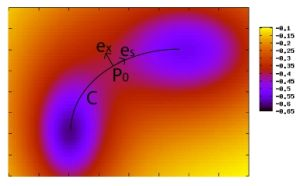Fig. 1: A typical potential energy surface of chemical reaction in a linear collision. The vectors used below is also shown. The equation used to generate this figure is z = -1/(5(x-1)^2 + (y-3)^2 + 2) - 1/((x-3)^2 + (y-1)^2 + 2).

Introduction

When chemical reaction in a linear collision of three molecules A+BC→AB+C is considered, two degrees of freedom, or two coordinates, are needed to describe the internal mechanics of the reaction, apart from one degree of freedom for the center-of-mass motion. If we imagine a 2-dimensional space spanned by the two coordinates, then the chemical reaction is understood by an imaginary particle motion in the space under some potential due to the interaction between the molecules. In this report, we introduce an attempt to analyze such motion with vibrationally adiabatic approximation by classical mechanics .

The outline of this report is as follows: we first describe the qualitative feature of a particular type of potential energy we want to discuss; next, the Hamilton-Jacobi equation is constructed after the coordinate system suitable for the potential energy surface of the problem is briefly explained; we then solve the equation by separating variables and using adiabatic approximation for vibrational motion. Even though the adiabatic approximation is not valid for many actual cases, we focus on the adiabatic limit for simplicity.

Potential Energy Surface of Interest

The potential energy surface in the 2-dimensional space obtained in the procedure described above typically has following features. First of all, there should be two local minima, each corresponding to reactants (A+BC) and products (AB+C). Secondly, there is a relatively low energy smooth path (we call it C) which connects the reactants state with the products state. The path C is usually called "reaction path" in the literature, and the maximum energy state in C is identified as the transition state of the chemical reaction. Finally, states along C are stable against perturbation in the direction perpendicular to C and a particle's motion in this direction is thus oscillatory.

Figure 1 is an example of a contour plot of potential surface geometry. Note that the Fig. 1 merely serves to explain the features listed above and does not represent potential energy surface of any real chemical reaction .

In a realistic calculation, the potential energy surface is often plotted by using skewed coordinate axes. This is in order to simplify the kinetic energy by removing a cross term which exists in center-of-mass Cartesian coordinates in addition to the normal terms proportional to the square of each momentum. Moreover, the mass along each axis can become the same by the choice of the skewed axis . We therefore should emphasize that the calculation in this report does not assume Cartesian coordinate system in the potential energy surface plot. Note that the Fig. 1 was drawn with Cartesian coordinates just for ease.

Coordinate System along Curve C and Hamilton-Jacobi Equation

Since we expect that the motion of the imaginary particle in the 2-D space is confined only to the vicinity of Curve C, the coordinate system along Curve C seems a natural choice. Specifically, the new coordinate system is constructed by the following procedure: we first find the point P0 which is on C and the closest to the point P of the particle; the distance x between P and P0 is the one of the new coordinates; the length s along C from a fixed point OC to P0 is the other one. This coordinate system (x, s) is in fact very convenient in order to separate variables and apply adiabatic approximations, which will be explained later. In addition, by transforming the initail coordinate system into the coordinate system along C, we no longer need to worry about the initial (possibly skewed) coordinate system.

Before proceeding further, let us explain the notations used in the following discussion. The vector from the point of origin O to P is r(s), and the vector from O to P0is r0(s). The unit vector pointing from P0 toward P is ex(s) and the unit vector from P0 along C is es(s) (see also Fig. 1). The relations between these vectors are listed below: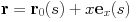(1)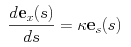(2)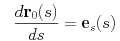(3)

where κ is the curvature of C at P0. The equation (2) follows directly from the definition of curvature or from the Serret-Frenet formula in elementary differential geometry .

What we need next in order to write down Hamiltonian is the canonical momentum of the new coordinate system. We use the contact transformation method by the generating function ψ′ = pr to obtain the momentum . By taking the derivative of ψ′ with respect to coordinates, it is shown that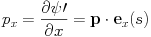(4)

and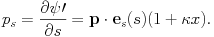(5)

On the other hand, it is convenient to separate the potential energy V into two parts V1(s) and V2(x, s). V1(s) is defined as the potential along C, while V2(x, s) ≡ V - V1. V2(0, s) =0 due to the definition of V1(s).

Now we are ready to write down the Hamiltonian as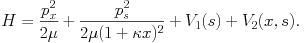(6)

This Hamiltonian immediately leads to the Hamilton-Jacobi equation ;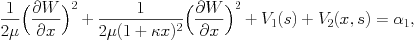(7)

where W(x, s, α) is the Hamilton's characteristic function and α1 is a constant of motion representing the total energy. The momenta are related to W by the following equations: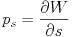(8)

and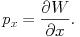(9)

Separation of Variables

The equation (7) is of course not easy to solve because it involves two coordinates (x and s). We therefore try to separate the variables in this section. Specifically we seek for a solution in the following form: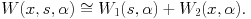(10)

The reason why we used "≅" instead of "=" is simply because, as we will see later, without adiabatic approximation we cannot obtain the solution in the form (10).

After substitution of (10) into (7) and some algebra, the equation (7) becomes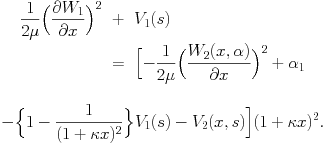(11)

In the equation (11), we immediately see that the left-hand side does not contain x, which allows us to set the both sides equal to a function α2(s) of only s. Thus (11) becomes the following two equations: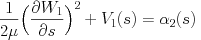(12)

and(13)

where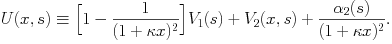(14)

The two equations (12) and (13) appear to be two separate Hamilton-Jacobi equations, one (12) for s and the other (13) for x, except for the fact that (13) includes s. (12) corresponds to the motion of s along C, while (13) represents the vibrational motion of x in a direction perpendicular to C. From this point of view, U(x, s) serves as an effective potential for the vibrational motion.

The physical meaning of the adiabatic approximation is to assume that the time scale of x, that is, the period of the oscillatory motion is much faster than the time scale of s, that is, the motion along C. With this approximation, the slow motion of s is influenced not directly by the fast oscillatory motion of x but only by the time average of x. This is the reason why (12) does not explicitly include x.

The consideration in the previous paragraph implies what we should do next. We need to calculate the x0 which minimizes U(x, s). Since x0 can be looked on as the average, or the center, of x through vibrational motion, we can expand functions of x including U(x, s) around x = x0. After the expansion, we can effectively average out the vibrational motion and will obtain an essential equation to determine α(s).

Let us follow the procedure above. The derivative of (14) with respect to x should be zero at x = x0, so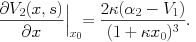(15)

By solving (15) with respect to x0, we obtain x0 using α2. We next expand functions of x in (11) around x0 and, by using (8) and (14), we have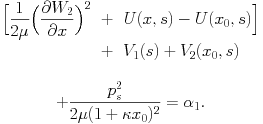(16)

The equation (16) is very convenient because x is not contained except in the first bracket, which represents vibrational energy. We can then solve the equation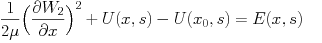(17)

and take the average of E over the time scale longer than the period of vibration in order to obtain E0. This E0 is substituted into (16), giving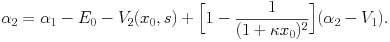(18)

Note that introducing an action variable for the vibrational motion makes it easier to obtain E0, though it is not essential. This procedure is explained in .

Procedure to Predict the Time Evolution of S

Since we have all the essential equations, we here summarize the solution procedure of the standard problem in which s is to be determined as a function of t given V1, V2 and initial conditions. We have already noted that (15) gives the functional form of x0(s) by using α2. This x0 can be substituted into (18) and we can determine α2(s). (12) then becomes very easy integration problem and W1(s, α) is thus obtained. Finally, W1 is substituted into (8) to give the relation between momentum ps and coordinate s. Since Hamilton's equation suggests that ps = μ(1 + κx0)ds/dt, s(t) is thus determined.

© 2007 Ko Munakata. The author grants permission to copy, distribute and display this work in unaltered form, with attributation to the author, for noncommercial purposes only. All other rights, including commercial rights, are reserved to the author.

References

 R. A. Marcus, J. Chem. Phys. 45, 4500 (1966).

 Potential energy surface plots obtained by simulating realistic systems are found in many articles about kinetic theory of chemical reaction. See, for example, Fig. 3 in R. E. Weston, J. Chem. Phys. 31, 892 (1959).

 C. G. Gibson, Elementary Geometry of Differentiable Curves: An Undergraduate Introduction (Cambridge, 2001).

 H. C. Corben and P. Stehle, Classical Mechanics (Wiley, 1960). Section 58 and 59 of this book treat various contact transformation methods by generating function, while sec. 95 explains a topic similar to the discussion leading to the Hamiltonian (6).

 H. Goldstein, C. Pole and J. Safko, Classical Mechanics (Addison-Wesley, 2002).